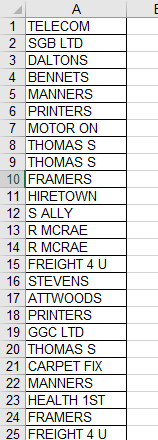# VBA – Populate Array with Unique Values from Column

This tutorial will teach you how to populate an array with unique values from a column in VBA.

## Populate Array with Unique Values from Column

Taking a list of values from an Excel sheet into an Array is a matter of looping through the rows in  Excel and populating the array.

Consider the following list of customers in an Excel sheet.Using a loop, we could populate an Array in VBA. However, a standard loop will loop through each row, including duplicate rows and you will end up with duplicate values in your Array.

The solution to to loop through the cells and populate a Collection object with the values as a collection object will not allow duplicates. You can then use that collection object to populate your array.

``````Sub PopulateUniqueArray()
Dim StrCustomers() As String
Dim Col As New Collection
Dim valCell As String
Dim i As Integer
Dim n As Integer
'count the rows in the range
n = Range("A1", Range("A1").End(xlDown)).Rows.Count
'Populate Temporary Collection
On Error Resume Next
For i = 0 To n
valCell = Range("A1").Offset(i, 0).Value
Next i
Err.Clear
On Error GoTo 0
'Resize n
n = Col.Count
'Redeclare array
ReDim StrCustomers(1 To n)
'Populate Array by looping through the collection
For i = 1 To Col.Count
StrCustomers(i) = Col(i)
Next i
Debug.Print Join(StrCustomers(), vbCrLf)
End Sub``````

Populate Array w/ Unique Values – Function

The above example showed a procedure that would perform your desired actions. But instead you might prefer a portable Function to perform the task:

``````Function CreateUniqueList(nStart as Long, nEnd as long) as Variant
Dim Col As New Collection
Dim arrTemp() As String
Dim valCell As String
Dim i As Integer
'Populate Temporary Collection
On Error Resume Next
For i = 0 To nEnd
valCell = Range("A" & nStart).Offset(i, 0).Value
Next i
Err.Clear
On Error GoTo 0
'Resize n
nEnd = Col.Count
'Redeclare array
ReDim arrTemp(1 To nEnd )
'Populate temporary array by looping through the collection
For i = 1 To Col.Count
arrTemp(i) = Col(i)
Next i
'return the temporary array to the function result
CreateUniqueList = arrTemp()
End Function``````

To use the Function, you’ll need to call it from a Sub Procedure:

``````Sub PopulateArray()
Dim StrCustomers() As String
Dim strCol as Collection
Dim n As Long
'count the rows in the range
n = Range("A1", Range("A1").End(xlDown)).Rows.Count
'run the function to create an array of unique values
strCustomers() = CreateUniqueList(1,n)
End Sub
``````

Stop searching for VBA code online. Learn more about AutoMacro - A VBA Code Builder that allows beginners to code procedures from scratch with minimal coding knowledge and with many time-saving features for all users!